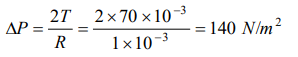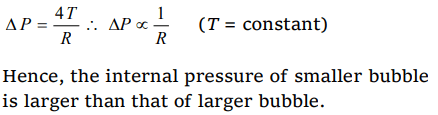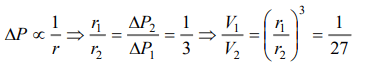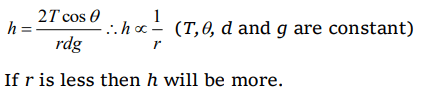## Mechanical Properties of Fluids Questions and Answers Part-13

1.A spherical drop of water has radius 1 mm If surface tension of water is 70 * 10-3 N/m difference of pressures between inside and out side of the spherical drop is
a) $35 N\diagup m^{2}$
b) $70 N\diagup m^{2}$
c) $140 N\diagup m^{2}$
d) Zero

Explanation:2. The pressure at the bottom of a tank containing a liquid does not depend on
a) Acceleration due to gravity
b) Height of the liquid column
c) Area of the bottom surface
d) Nature of the liquid

Explanation:3. In capillary pressure below the curved surface of water will be
a) Equal to atmospheric
b) Equal to upper side pressure
c) More than upper side pressure
d) Lesser than upper side pressure

Explanation: Lesser than upper side pressure

4. Two soap bubbles of radii $r_{1}$ and $r_{2}$ equal to 4 cm and 5 cm are touching each other over a common surface $S_{1}S_{2}$ (shown in figure). Its radius will bea) 4 cm
b) 20 cm
c) 5 cm
d) 4.5 cm

Explanation:5. The pressure inside a small air bubble of radius 0.1 mm situated just below the surface of water will be equal to [ Take surface tension of water $70\times10^{-3}Nm^{-1}$   and atmospheric pressure = $1.013\times10^{5}Nm^{-2}$   ]
a) $2.054\times10^{3}Pa$
b) $1.027\times10^{3}Pa$
c) $1.027\times10^{5}Pa$
d) $2.054\times10^{5}Pa$

Explanation:6. Two bubbles A and B $\left(A>B\right)$  are joined through a narrow tube. Then
a) The size of A will increase
b) The size of B will increase
c) The size of B will increase until the pressure equals
d) None of these

Explanation:7. Two soap bubbles have different radii but their surface tension is the same. Mark the correct statement
a) Internal pressure of the smaller bubble is higher than the internal pressure of the larger bubble
b) Pressure of the larger bubble is higher than the smaller bubble
c) Both bubbles have the same internal pressure
d) None of the above

Explanation:8. If the excess pressure inside a soap bubble is balanced by oil column of height 2 mm, then the surface tension of soap solution will be (r = 1 cm and density d = 0.8 gm/cc)
a) 3.9 N/m
b) $3.9 ×10^{-2} N/m$
c) $3.9 ×10^{-3} N/m$
d) 3.9 dyne/m

Explanation:9. The excess pressure in a soap bubble is thrice that in other one. Then the ratio of their volume is
a) 1 : 3
b) 1 : 9
c) 27 : 1
d) 1 : 27